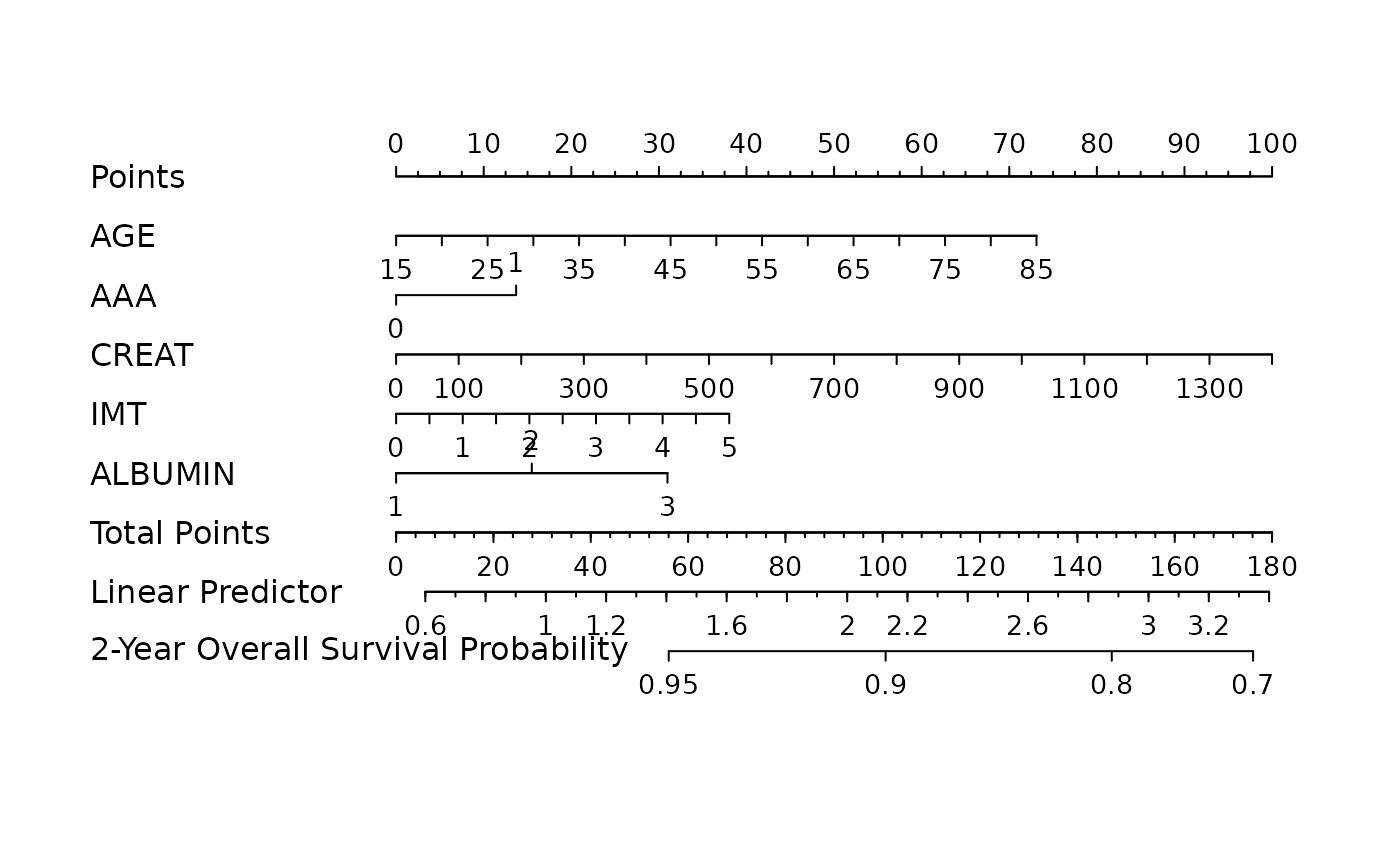Automatic model selection for high-dimensional Cox models with Mnet penalty, evaluated by penalized partial-likelihood.

fit_mnet(x, y, nfolds = 5L, gammas = c(1.01, 1.7, 3, 100),
alphas = seq(0.05, 0.95, 0.05), eps = 1e-04, max.iter = 10000L,
seed = 1001, trace = FALSE, parallel = FALSE)

## Arguments

x Data matrix. Response matrix made by Surv. Fold numbers of cross-validation. Gammas to tune in cv.ncvsurv. Alphas to tune in cv.ncvsurv. Convergence threshhold. Maximum number of iterations. A random seed for cross-validation fold division. Output the cross-validation parameter tuning progress or not. Default is FALSE. Logical. Enable parallel parameter tuning or not, default is FALSE. To enable parallel tuning, load the doParallel package and run registerDoParallel() with the number of CPU cores before calling this function.

## Examples

data("smart")
x <- as.matrix(smart[, -c(1, 2)])[1:120, ]
time <- smart$TEVENT[1:120] event <- smart$EVENT[1:120]
y <- survival::Surv(time, event)

fit <- fit_mnet(
x, y,
nfolds = 3,
gammas = 3, alphas = c(0.3, 0.8),
max.iter = 15000, seed = 1010
)

nom <- as_nomogram(
fit, x, time, event,
pred.at = 365 * 2,
funlabel = "2-Year Overall Survival Probability"
)

plot(nom)